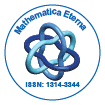# Mathematica EternaOpen Access

ISSN: 1314-3344

## Abstract

### Formulas of Fredholm Type for Fredholm Linear Equations in Fr´echet Spaces

Gra ÃÂzyna Ciecierska

We consider Fredholm bounded linear operators S+T acting from one Fr´echet space X into another one Y , where S is Fredholm and T is nuclear. We obtain formulas for solutions of the induced equations: (S + T)x = y0, y ∗ (S + T) = x ∗ 0 . These formulas are abstract analogues of the classical formulas for solutions of Fredholm integral equations in the space of continuous functions on [a, b]. In this approach the main tools are provided by the theory of determinant systems. The effective formulas for determinant systems for nuclear perturbations of Fredholm operators in Fr´echet spaces play the crucial role and lead to formulas of Fredholm type.

Top